Improving Performance – Part One: Asynchronous/Synchronous Operations in Acumatica

# Improving Performance – Part One: Asynchronous/Synchronous Operations in Acumatica

Yuriy Zaletskyy | June 30, 2023

## Introduction

In this two-part series blog post, I want to share with you all how asynchronous/synchronous operations & multithreading work within the Acumatica framework using C#. I will relate how you can improve performance – what works & what doesn’t, as well as how caching may help you to improve performance out of the box without requiring multi-threading optimizations in your code.  First up is Synchronous & Asynchronous operations.  I’ll cover multithreading in my next post which will be published in a couple of days.

## Synchronous & Asynchronous Operations

Quite often there is a need to create custom inquiries that are based on some sophisticated aggregations in the database. Suppose that you have a task to calculate the totals of all sales orders in the database for Ordered Qty, Order Total, and Tax Total.  You can implement it in a way that initially is synchronous, then asynchronous as follows:

``````

public PXAction<SOOrder> DifferentTests;

[PXButton]
[PXUIField(DisplayName = "Async test")]
{
PXLongOperation.StartOperation(Base, delegate
{
ExecuteTests();
});

}

private void ExecuteTests()
{
var sw = new Stopwatch();
sw.Start();

var r1 = GetAllCuryOrderTotal1();
var r2 = GetAllCuryTaxTotalTotal1();
var r3 = GetAllOrderQty1();

sw.Stop();

PXTrace.WriteInformation(\$"Milliseconds passed for sync: {sw.ElapsedMilliseconds}, r1 ={r1}, r2={r2}, r3 = {r3}");

var sw1 = new Stopwatch();
sw1.Start();
var t1 = GetAllCuryOrderTotal2();
var t2 = GetAllCuryTaxTotalTotal2();
var t3 = GetAllOrderQty2();

sw1.Stop();
PXTrace.WriteInformation(\$"Milliseconds passed for async: {sw1.ElapsedMilliseconds}, r1 ={t1.Result}, " +
\$"r2={t2.Result}, r3 = {t3.Result}");
}

public decimal GetAllCuryOrderTotal1()
{
decimal sum = 0.0m;

foreach (var soOrder in PXSelect<SOOrder>.Select(Base).ToList())
{
sum += soOrder.GetItem<SOOrder>().CuryOrderTotal ?? 0.0m;
}
return sum;
}

public decimal GetAllCuryTaxTotalTotal1()
{
decimal sum = 0.0m;

foreach (var soOrder in PXSelect<SOOrder>.Select(Base).ToList())
{
sum += soOrder.GetItem<SOOrder>().CuryTaxTotal ?? 0.0m;
}
return sum;
}

public decimal GetAllOrderQty1()
{
decimal sum = 0.0m;

foreach (var soOrder in PXSelect<SOOrder>.Select(Base).ToList())
{
sum += soOrder.GetItem<SOOrder>().OrderQty ?? 0.0m;
}
return sum;
}

{
decimal sum = 0.0m;
() =>
{

foreach (var soOrder in PXSelect<SOOrder>.Select(Base).ToList())
{
sum += soOrder.GetItem<SOOrder>().CuryOrderTotal ?? 0.0m;
}
}
);
return sum;
}

{
decimal sum = 0.0m;
() =>
{

foreach (var soOrder in PXSelect<SOOrder>.Select(Base).ToList())
{
sum += soOrder.GetItem<SOOrder>().CuryTaxTotal ?? 0.0m;
}
}
);
return sum;
}

{
decimal sum = 0.0m;
() =>
{

foreach (var soOrder in PXSelect<SOOrder>.Select(Base).ToList())
{
sum += soOrder.GetItem<SOOrder>().OrderQty ?? 0.0m;
}
}
);
return sum;
}

``````

Then, as you can see in screen-shot of the trace window the following details: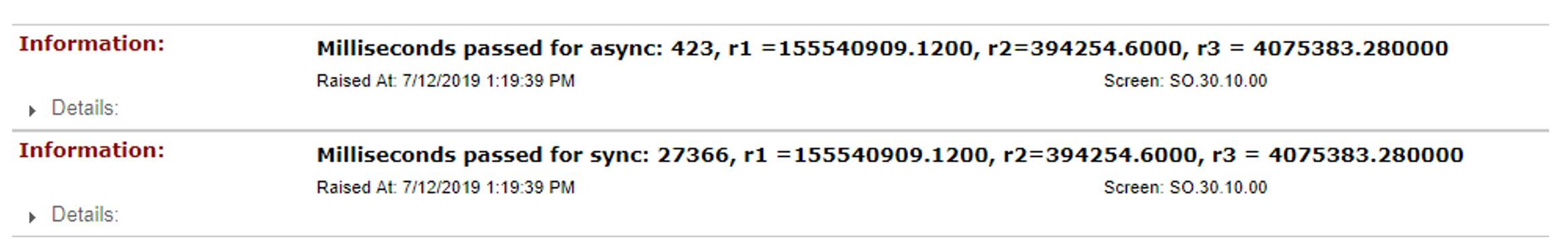Note that the synchronous version took 27,366 ms to run, and asynchronous code only 423 ms (64 times faster). It would appear that it would be a good idea to re-write our custom inquiries for asynchronous versions of our code. However, don’t be fooled as that would be bad idea. In the code fragment below, I think you will understand why:

``````
private void ExecuteTests()
{
foreach (var soOrder in PXSelect<SOOrder>.Select(Base).ToList())
{
}

var sw = new Stopwatch();
sw.Start();

var r1 = GetAllCuryOrderTotal1();
``````

Here is what we see in the trace window:The sync version took 27,366 ms to run, and async only 423 (64 times faster). Looks like it is time to re-write custom inquiries for our async version. But don’t rush to conclusions, as this is a mistake. I think the code fragment below will be self-explanatory:

``````
private void ExecuteTests()
{
foreach (var soOrder in PXSelect<SOOrder>.Select(Base).ToList())
{
}

var sw = new Stopwatch();
sw.Start();

var r1 = GetAllCuryOrderTotal1();

``````

The rest of the code is the same as before, but take a note of the results: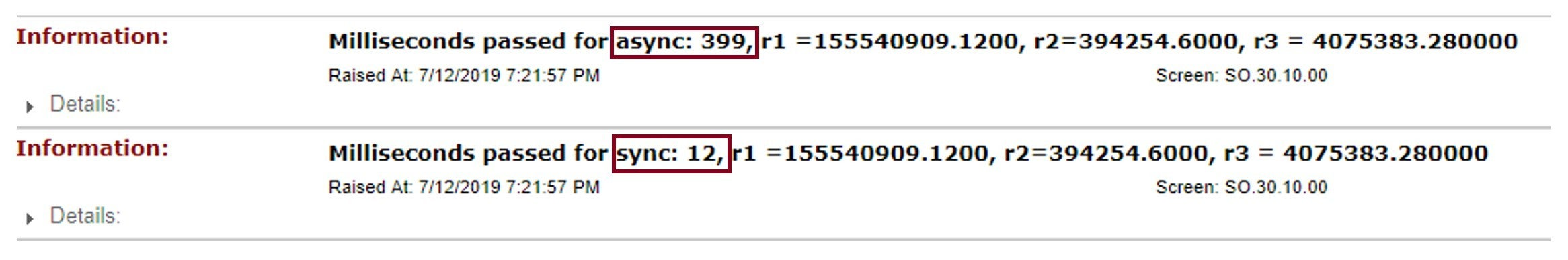Surprising results  to be sure.  The synchronous summation took only 12 milliseconds, while asynchronous took 399 milliseconds! The reason behind such an improvement is Acumatica’s caching mechanism. The initial Foreach that had enumerated all sales orders put those sales orders in Acumatica’s cache and perhaps SQL server did some caching as well here.  The outcome of synchronous summation took only 12 milliseconds instead initial 27,366.

Thus, one of take home points here could be re-enumeration of records can improve performance.  This is because it places records in the cache and eliminates any round trips to the database. Or, if you want to be sure, just read all the records from the database into some piece of memory, and execute the calculations there.

By the way, if you are unlucky, you can get an error message: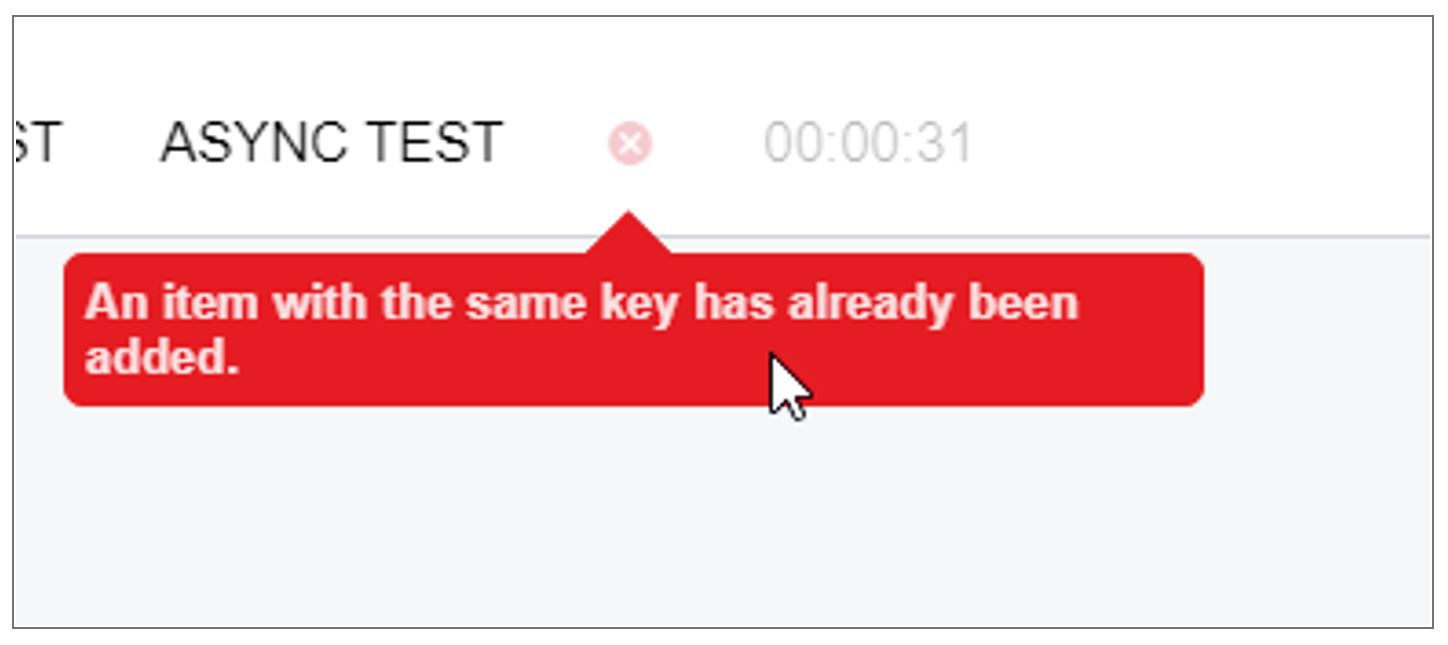Without digging into the details, this error message is caused by the fact that Acumatica graphs by default are not thread safe. Therefore, if you want to avoid such error messages, you’ll need to modify any async methods you may have in the following way:

``````
{
decimal sum = 0.0m;
() =>
{
var gr = PXGraph.CreateInstance<SOOrderEntry>();
foreach (var soOrder in PXSelect<SOOrder>.Select(gr).ToList())
{
sum += soOrder.GetItem<SOOrder>().CuryOrderTotal ?? 0.0m;
}
}
);
return sum;
}
``````

The basic idea here is that the change for each thread will get it’s own graph,  and thus threads will not cause collisions in your code. I thought I would add a graph creation to the sync code, and here are the results of the stack trace I observed: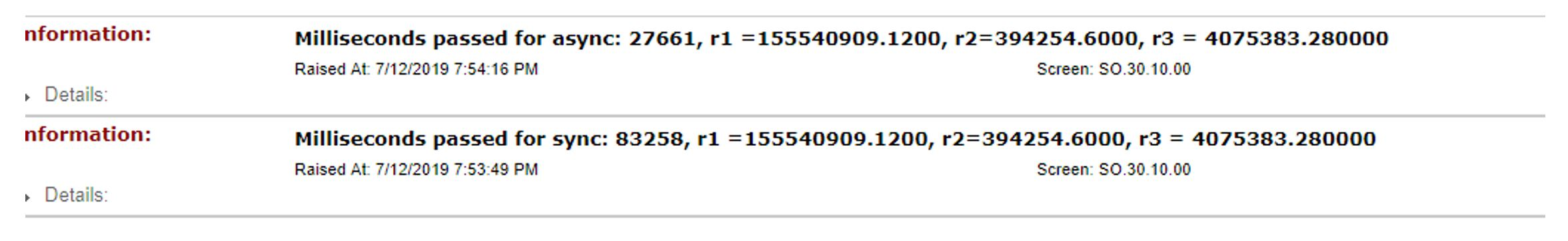You observe that with a separate graph creation (async vs sync) we notice that async is faster – but there is one issue. The sync code version doesn’t need such “optimization”. I introduced it just to show you a fair apples to apples comparison.

Here’s the full source code of this approach:

``````
using System;
using System.Collections;
using System.Collections.Generic;
using System.Diagnostics;
using System.Linq;
using System.Text;
using PX.Data;
using PX.Objects.SO;

{
public class SOOrderEntryExt : PXGraphExtension<SOOrderEntry>
{

[PXButton]
{

}

public PXAction<SOOrder> DifferentTests;

[PXButton]
[PXUIField(DisplayName = "Async test")]
{
PXLongOperation.StartOperation(Base, delegate
{
ExecuteTests();
});

}

private void ExecuteTests()
{
foreach (var soOrder in PXSelect<SOOrder>.Select(Base).ToList())//this foreach intended for eliminating effect of caching of records in Acumatica
{
}

var sw = new Stopwatch();
sw.Start();

var r1 = GetAllCuryOrderTotal1();
var r2 = GetAllCuryTaxTotalTotal1();
var r3 = GetAllOrderQty1();

sw.Stop();

PXTrace.WriteInformation(\$"Milliseconds passed for sync: {sw.ElapsedMilliseconds}, r1 ={r1}, r2={r2}, r3 = {r3}");

var sw1 = new Stopwatch();
sw1.Start();

var t1 = GetAllCuryOrderTotal2();
var t2 = GetAllCuryTaxTotalTotal2();
var t3 = GetAllOrderQty2();

sw1.Stop();
PXTrace.WriteInformation(\$"Milliseconds passed for async: {sw1.ElapsedMilliseconds}, r1 ={t1.Result}, " +
\$"r2={t2.Result}, r3 = {t3.Result}");
}

public decimal GetAllCuryOrderTotal1()
{
decimal sum = 0.0m;
var gr = PXGraph.CreateInstance<SOOrderEntry>();
foreach (var soOrder in PXSelect<SOOrder>.Select(gr).ToList())
{
sum += soOrder.GetItem<SOOrder>().CuryOrderTotal ?? 0.0m;
}
return sum;
}

public decimal GetAllCuryTaxTotalTotal1()
{
decimal sum = 0.0m;
var gr = PXGraph.CreateInstance<SOOrderEntry>();
foreach (var soOrder in PXSelect<SOOrder>.Select(gr).ToList())
{
sum += soOrder.GetItem<SOOrder>().CuryTaxTotal ?? 0.0m;
}
return sum;
}

public decimal GetAllOrderQty1()
{
decimal sum = 0.0m;
var gr = PXGraph.CreateInstance<SOOrderEntry>();
foreach (var soOrder in PXSelect<SOOrder>.Select(gr).ToList())
{
sum += soOrder.GetItem<SOOrder>().OrderQty ?? 0.0m;
}
return sum;
}

{
decimal sum = 0.0m;
() =>
{
var gr = PXGraph.CreateInstance<SOOrderEntry>();
foreach (var soOrder in PXSelect<SOOrder>.Select(gr).ToList())
{
sum += soOrder.GetItem<SOOrder>().CuryOrderTotal ?? 0.0m;
}
}
);
return sum;
}

{
decimal sum = 0.0m;
() =>
{
var gr = PXGraph.CreateInstance<SOOrderEntry>();
foreach (var soOrder in PXSelect<SOOrder>.Select(gr).ToList())
{
sum += soOrder.GetItem<SOOrder>().CuryTaxTotal ?? 0.0m;
}
}
);
return sum;
}

{
decimal sum = 0.0m;
() =>
{
var gr = PXGraph.CreateInstance<SOOrderEntry>();
foreach (var soOrder in PXSelect<SOOrder>.Select(gr).ToList())
{
sum += soOrder.GetItem<SOOrder>().OrderQty ?? 0.0m;
}
}
);
return sum;
}
}
}

``````

A logical question one might ask:  how do we achieve one graph for sync totals calculations, and one graph for async calculations. After some thought, I came up with the following code:

``````
using System;
using System.Collections;
using System.Collections.Generic;
using System.Diagnostics;
using System.Linq;
using System.Text;
using PX.Data;
using PX.Objects.SO;

{
public class SOOrderEntryExt : PXGraphExtension<SOOrderEntry>
{

[PXButton]
{

}

public PXAction<SOOrder> DifferentTests;

[PXButton]
[PXUIField(DisplayName = "Async test")]
{
PXLongOperation.StartOperation(Base, delegate
{
ExecuteTests();
});

}

private void ExecuteTests()
{
int numberOfIterations = 100;

foreach (var soOrder in PXSelect<SOOrder>.Select(Base).ToList())//this foreach intended for eliminating effect of caching of records in Acumatica
{
}

var sw = new Stopwatch();
sw.Start();
decimal r1, r2, r3;

for(int i = 0; i < numberOfIterations; i++)
{
r1 = GetAllCuryOrderTotal1();
r2 = GetAllCuryTaxTotalTotal1();
r3 = GetAllOrderQty1();
}

sw.Stop();

PXTrace.WriteInformation(\$"Milliseconds passed for sync: {sw.ElapsedMilliseconds}, r1 ={r1}, r2={r2}, r3 = {r3}");

var sw1 = new Stopwatch();
sw1.Start();

Task<decimal> t1 = null, t2 = null, t3 = null;

var g1 = PXGraph.CreateInstance<SOOrderEntry>();
var g2 = PXGraph.CreateInstance<SOOrderEntry>();
var g3 = PXGraph.CreateInstance<SOOrderEntry>();

for (int i = 0; i < numberOfIterations; i++)
{
t1 = GetAllCuryOrderTotal2(g1);
t2 = GetAllCuryTaxTotalTotal2(g2);
t3 = GetAllOrderQty2(g3);

}

sw1.Stop();
PXTrace.WriteInformation(\$"Milliseconds passed for async: {sw1.ElapsedMilliseconds}, r1 ={t1.Result}, " +
\$"r2={t2.Result}, r3 = {t3.Result}");
}

public decimal GetAllCuryOrderTotal1()
{
decimal sum = 0.0m;
foreach (var soOrder in PXSelect<SOOrder>.Select(Base).ToList())
{
sum += soOrder.GetItem<SOOrder>().CuryOrderTotal ?? 0.0m;
}
return sum;
}

public decimal GetAllCuryTaxTotalTotal1()
{
decimal sum = 0.0m;
foreach (var soOrder in PXSelect<SOOrder>.Select(Base).ToList())
{
sum += soOrder.GetItem<SOOrder>().CuryTaxTotal ?? 0.0m;
}
return sum;
}

public decimal GetAllOrderQty1()
{
decimal sum = 0.0m;

foreach (var soOrder in PXSelect<SOOrder>.Select(Base).ToList())
{
sum += soOrder.GetItem<SOOrder>().OrderQty ?? 0.0m;
}
return sum;
}

{
decimal sum = 0.0m;
() =>
{
foreach (var soOrder in PXSelect<SOOrder>.Select(gr).ToList())
{
sum += soOrder.GetItem<SOOrder>().CuryOrderTotal ?? 0.0m;
}
}
);
return sum;
}

{
decimal sum = 0.0m;
() =>
{
foreach (var soOrder in PXSelect<SOOrder>.Select(gr).ToList())
{
sum += soOrder.GetItem<SOOrder>().CuryTaxTotal ?? 0.0m;
}
}
);
return sum;
}

{
decimal sum = 0.0m;
() =>
{
foreach (var soOrder in PXSelect<SOOrder>.Select(gr).ToList())
{
sum += soOrder.GetItem<SOOrder>().OrderQty ?? 0.0m;
}
}
);
return sum;
}
}
}

``````

As you can see from the code, I have the base come back to the sync version, and each method has its own graph instance. Also, in order to imitate a large amount of data (sales demo database has only 3,348 sales orders ), I also introduced this per cycle.

And here are the results – 100 cycles (equal to 300,000 records):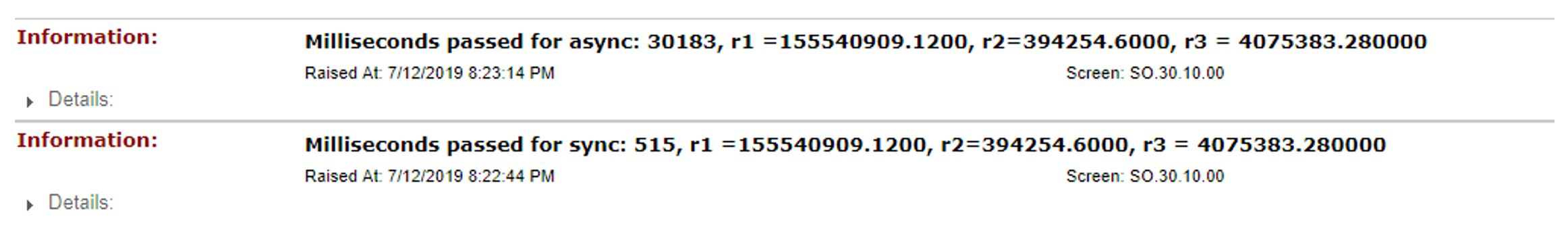1,000 cycles (equal to 3,000,000 records):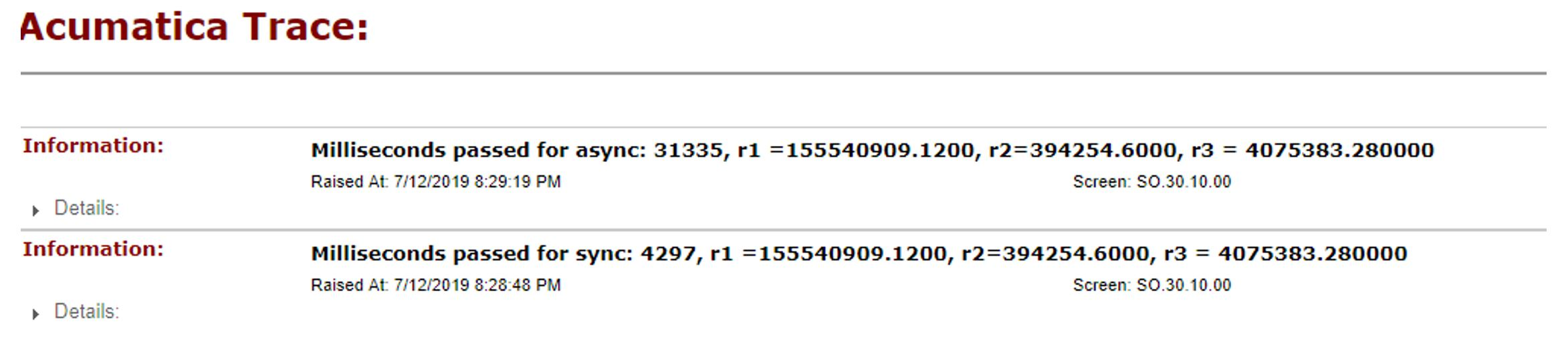Note that 100,000 cycles (equal to 30 million records):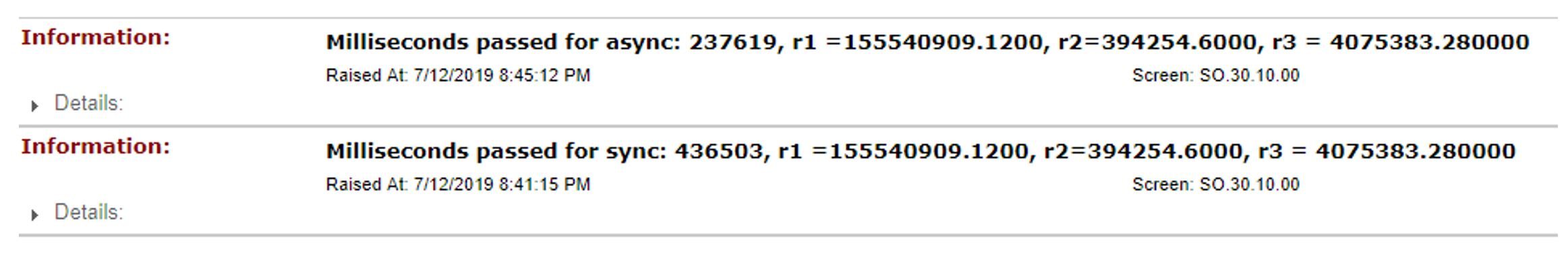As you can see from the screenshot, at 30 million records, the difference in performance between sync/async. In numeric representation this difference is 436503/237619 ≈ 1.837 In order to continue, I want to add some additional If conditions for making logic calculations more complex, and see if it will have any noticeable effect. Below gives a sample of of the changes I made:

``````
public bool IsMultipleOf2(string str)
{
try
{
char last = str[str.Length - 1];
int number = int.Parse(last.ToString());
return number % 2 == 0;
}
catch
{
return true;
}
}

public decimal GetAllCuryOrderTotal1()
{
decimal sum = 0.0m;
foreach (var soOrder in PXSelect<SOOrder>.Select(Base).ToList())
{
if (IsMultipleOf2(soOrder.GetItem<SOOrder>().OrderNbr))
{
sum += soOrder.GetItem<SOOrder>().CuryOrderTotal ?? 0.0m;
}
else
{
var number = soOrder.GetItem<SOOrder>().CuryOrderTotal ?? 0.0m;
sum += number * number;
}
}
return sum;
}
``````

As you can see from the code, I have added relatively small changes, but take a look what effect it has on performance difference: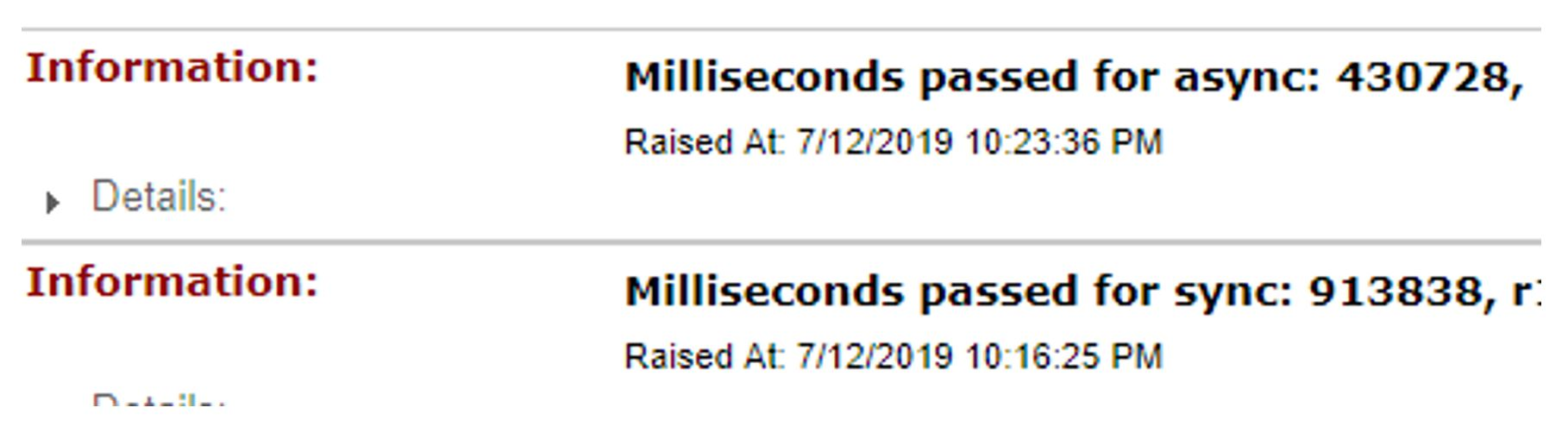913838 / 430728 ≈ 2.12

Here again is the full source code:

``````
using System;
using System.Collections;
using System.Collections.Generic;
using System.Diagnostics;
using System.Linq;
using System.Text;
using PX.Data;
using PX.Objects.SO;

{
public class SOOrderEntryExt : PXGraphExtension<SOOrderEntry>
{

[PXButton]
{

}

public PXAction<SOOrder> DifferentTests;

[PXButton]
[PXUIField(DisplayName = "Async test")]
{
PXLongOperation.StartOperation(Base, delegate
{
ExecuteTests();
});

}

private void ExecuteTests()
{
int numberOfIterations = 100000;

foreach (var soOrder in PXSelect<SOOrder>.Select(Base).ToList())//this foreach intended for eliminating effect of caching of records in Acumatica
{
}

var sw = new Stopwatch();
sw.Start();
decimal r1 = 0, r2 = 0, r3 = 0;

for(int i = 0; i < numberOfIterations; i++)
{
r1 = GetAllCuryOrderTotal1();
r2 = GetAllCuryTaxTotalTotal1();
r3 = GetAllOrderQty1();
}

sw.Stop();

PXTrace.WriteInformation(\$"Milliseconds passed for sync: {sw.ElapsedMilliseconds}, r1 ={r1}, r2={r2}, r3 = {r3}");

var sw1 = new Stopwatch();
sw1.Start();

Task<decimal> t1 = null, t2 = null, t3 = null;

var g1 = PXGraph.CreateInstance<SOOrderEntry>();
var g2 = PXGraph.CreateInstance<SOOrderEntry>();
var g3 = PXGraph.CreateInstance<SOOrderEntry>();

for (int i = 0; i < numberOfIterations; i++)
{
t1 = GetAllCuryOrderTotal2(g1);
t2 = GetAllCuryTaxTotalTotal2(g2);
t3 = GetAllOrderQty2(g3);

}

sw1.Stop();
PXTrace.WriteInformation(\$"Milliseconds passed for async: {sw1.ElapsedMilliseconds}, r1 ={t1.Result}, " +
\$"r2={t2.Result}, r3 = {t3.Result}");
}

public bool IsMultipleOf2(string str)
{
try
{
char last = str[str.Length - 1];
int number = int.Parse(last.ToString());
return number % 2 == 0;
}
catch
{
return true;
}
}

public decimal GetAllCuryOrderTotal1()
{
decimal sum = 0.0m;
foreach (var soOrder in PXSelect<SOOrder>.Select(Base).ToList())
{
if (IsMultipleOf2(soOrder.GetItem<SOOrder>().OrderNbr))
{
sum += soOrder.GetItem<SOOrder>().CuryOrderTotal ?? 0.0m;
}
else
{
var number = soOrder.GetItem<SOOrder>().CuryOrderTotal ?? 0.0m;
sum += number * number;
}
}
return sum;
}

public decimal GetAllCuryTaxTotalTotal1()
{
decimal sum = 0.0m;
foreach (var soOrder in PXSelect<SOOrder>.Select(Base).ToList())
{
if (IsMultipleOf2(soOrder.GetItem<SOOrder>().OrderNbr))
{
sum += soOrder.GetItem<SOOrder>().CuryTaxTotal ?? 0.0m;
}
else
{
var number = soOrder.GetItem<SOOrder>().CuryTaxTotal ?? 0.0m;
sum += number * number;
}
}
return sum;
}

public decimal GetAllOrderQty1()
{
decimal sum = 0.0m;

foreach (var soOrder in PXSelect<SOOrder>.Select(Base).ToList())
{
if (IsMultipleOf2(soOrder.GetItem<SOOrder>().OrderNbr))
{
sum += soOrder.GetItem<SOOrder>().OrderQty ?? 0.0m;
}
else
{
var number = soOrder.GetItem<SOOrder>().OrderQty ?? 0.0m;
sum += number * number;
}
}
return sum;
}

{
decimal sum = 0.0m;
() =>
{
foreach (var soOrder in PXSelect<SOOrder>.Select(gr).ToList())
{
if (IsMultipleOf2(soOrder.GetItem<SOOrder>().OrderNbr))
{
sum += soOrder.GetItem<SOOrder>().CuryOrderTotal ?? 0.0m;
}
else
{
var number = soOrder.GetItem<SOOrder>().CuryOrderTotal ?? 0.0m;
sum += number * number;
}
}
}
);
return sum;
}

{
decimal sum = 0.0m;
() =>
{
foreach (var soOrder in PXSelect<SOOrder>.Select(gr).ToList())
{
if (IsMultipleOf2(soOrder.GetItem<SOOrder>().OrderNbr))
{
sum += soOrder.GetItem<SOOrder>().CuryTaxTotal ?? 0.0m;
}
else
{
var number = soOrder.GetItem<SOOrder>().CuryTaxTotal ?? 0.0m;
sum += number * number;
}
}
}
);
return sum;
}

{
decimal sum = 0.0m;
() =>
{
foreach (var soOrder in PXSelect<SOOrder>.Select(gr).ToList())
{
if (IsMultipleOf2(soOrder.GetItem<SOOrder>().OrderNbr))
{
sum += soOrder.GetItem<SOOrder>().OrderQty ?? 0.0m;
}
else
{
var number = soOrder.GetItem<SOOrder>().OrderQty ?? 0.0m;
sum += number * number;
}
}
}
);
return sum;
}
}
}
``````

With just some additional logic, you can see this gives you the performance difference in time of execution of the sync/async version with the markedly improving the async version of the code.

## Summary

In this blog post, I’ve described one of two ways of speeding up performance using asynchronous tasks.  In Part Two, I will be illustrating some multitasking/multi-threading approaches. Both of these approaches can improve performance significantly, but not in 100%  of the cases. Real performance boosts will only gained if you have significantly large amounts of data to import, manipulate or massage.  What we are talking about here is data in the millions of records.

## Blog Author

Yuriy started programming in 2003 using C++ and FoxPro then switching to .Net in 2006. Beginning in 2013, he has been actively developing applications using the Acumatica xRP Framework, developing solutions for many clients over the years. He has a personal blog, aptly named Yuriy Zaletskyy’s Blog, where he has been documenting programming issues he’s run into over the past six years – sharing his observations and solutions freely with other Acumatica developers.
Categories: Developers